Updating search results...

# 37 Results

View
Selected filters:
• CCSS.Math.Practice.MP.8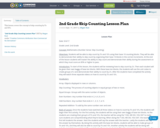Only Sharing Permitted
CC BY-NC-ND
Rating
0.0 stars

This lesson covers the concept of skip counting by 5's and 10's.

Subject:
Mathematics
Material Type:
Lesson
09/11/2017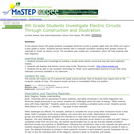Conditional Remix & Share Permitted
CC BY-NC-SA
Rating
0.0 stars

As an activity related to FOSS unit Magnetism and Electricity, 4th grade science students use a computer download to explore electrical circuits and to generate illustrations of electrical circuits for physical models built in class

Subject:
Career and Technical Education
Electronic Technology
Material Type:
Activity/Lab
Provider:
Science Education Resource Center (SERC) at Carleton College
Provider Set:
Pedagogy in Action
Author:
Lorraine Aaland
08/16/2012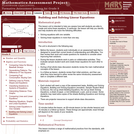Only Sharing Permitted
CC BY-NC-ND
Rating
0.0 stars

This lesson unit is intended to help teachers assess how well students are able to create and solve linear equations. In particular, the lesson will help you identify and help students who have the following difficulties: solving equations with one variable and solving linear equations in more than one way.

Subject:
Algebra
Mathematics
Material Type:
Assessment
Lesson Plan
Provider:
Shell Center for Mathematical Education
Provider Set:
Mathematics Assessment Project (MAP)
04/26/2013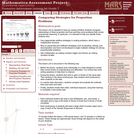Only Sharing Permitted
CC BY-NC-ND
Rating
0.0 stars

This lesson unit is intended to help you assess whether students recognize relationships of direct proportion and how well they solve problems that involve proportional reasoning. In particular, it is intended to help you identify those students who: use inappropriate additive strategies in scaling problems, which have a multiplicative structure; rely on piecemeal and inefficient strategies such as doubling, halving, and decomposition, and have not developed a single multiplier strategy for solving proportionality problems; and see multiplication as making numbers bigger, and division as making numbers smaller.

Subject:
Mathematics
Numbers and Operations
Material Type:
Assessment
Lesson Plan
Provider:
Shell Center for Mathematical Education
Provider Set:
Mathematics Assessment Project (MAP)
04/26/2013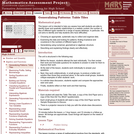Only Sharing Permitted
CC BY-NC-ND
Rating
0.0 stars

This lesson unit is intended to help teachers assess how well students are able to identify linear and quadratic relationships in a realistic context: the number of tiles of different types that are needed for a range of square tabletops. In particular, this unit aims to identify and help students who have difficulties with: choosing an appropriate, systematic way to collect and organize data; examining the data and looking for patterns; finding invariance and covariance in the numbers of different types of tile; generalizing using numerical, geometrical or algebraic structure; and describing and explaining findings clearly and effectively.

Subject:
Algebra
Geometry
Mathematics
Measurement and Data
Material Type:
Assessment
Lesson Plan
Provider:
Shell Center for Mathematical Education
Provider Set:
Mathematics Assessment Project (MAP)
04/26/2013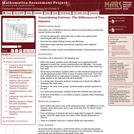Only Sharing Permitted
CC BY-NC-ND
Rating
0.0 stars

This lesson unit is intended to help you assess how well students working with square numbers are able to: choose an appropriate, systematic way to collect and organize data, examining the data for patterns; describe and explain findings clearly and effectively; generalize using numerical, geometrical, graphical and/or algebraic structure; and explain why certain results are possible/impossible, moving towards a proof.

Subject:
Algebra
Geometry
Mathematics
Measurement and Data
Material Type:
Assessment
Lesson Plan
Provider:
Shell Center for Mathematical Education
Provider Set:
Mathematics Assessment Project (MAP)
04/26/2013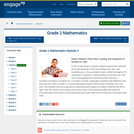Conditional Remix & Share Permitted
CC BY-NC-SA
Rating
0.0 stars

In this 25-day Grade 2 module, students expand their skill with and understanding of units by bundling ones, tens, and hundreds up to a thousand with straws. Unlike the length of 10 centimeters in Module 2, these bundles are discrete sets. One unit can be grabbed and counted just like a banana?1 hundred, 2 hundred, 3 hundred, etc. A number in Grade 1 generally consisted of two different units, tens and ones. Now, in Grade 2, a number generally consists of three units: hundreds, tens, and ones. The bundled units are organized by separating them largest to smallest, ordered from left to right. Over the course of the module, instruction moves from physical bundles that show the proportionality of the units to non-proportional place value disks and to numerals on the place value chart.

Find the rest of the EngageNY Mathematics resources at https://archive.org/details/engageny-mathematics.

Subject:
Mathematics
Numbers and Operations
Material Type:
Module
Provider:
New York State Education Department
Provider Set:
EngageNY
11/25/2012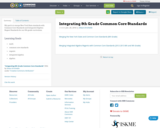Unrestricted Use
CC BY
Rating
0.0 stars

My goal is to merge New York State standards with Common Core Standards and Integrated Algebra Regent Standards for our 8th grade curriculum.

Subject:
Mathematics
Material Type:
Full Course
Lesson Plan
Syllabus
01/28/2016Unrestricted Use
CC BY
Rating
0.0 stars

Notice and Wonder is&nbsp;one of many Mathematically Productive Instructional Routines (MPIR).&nbsp;They&nbsp;are short (10ish minutes), daily exercises aimed at building&nbsp;number&nbsp;sense. This is one of six different MPIR covered in the&nbsp;Mathematically Productive Instructional Routines&nbsp;collection from the Washington Office of Public Instruction and the Washington Association of&nbsp;Educational Service Districts.

Subject:
Mathematics
Material Type:
Teaching/Learning Strategy
Author:
Barbara Soots
Washington OSPI OER Project
Washington OSPI Mathematics Department
04/08/2021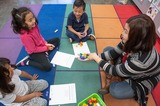Unrestricted Use
CC BY
Rating
0.0 stars

Number Talks are one of many Mathematically Productive Instructional Routines (MPIR).&nbsp;They&nbsp;are short (10ish minutes), daily exercises aimed at building&nbsp;number&nbsp;sense. This is one of six different MPIR covered in the Mathematically Productive Instructional Routines collection from the Washington Office of Public Instruction and the Washington Association of&nbsp;Educational Service Districts.

Subject:
Mathematics
Material Type:
Teaching/Learning Strategy
Author:
Barbara Soots
Washington OSPI Mathematics Department
Washington OSPI OER Project
04/08/2021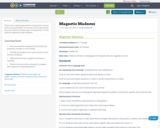Conditional Remix & Share Permitted
CC BY-NC-SA
Rating
0.0 stars

This is great exploring properties of magnetism science lab for young scientists. It is meant to be an experiential precursor before covering topics of magnetism. It could easily be adapted for older children.

Material Type:
Activity/Lab
01/28/2016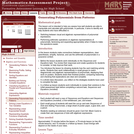Only Sharing Permitted
CC BY-NC-ND
Rating
0.0 stars

This lesson unit is intended to help you assess how well students are able to manipulate and calculate with polynomials. In particular, it aims to identify and help students who have difficulties in: switching between visual and algebraic representations of polynomial expressions; and performing arithmetic operations on algebraic representations of polynomials, factorizing and expanding appropriately when it helps to make the operations easier.

Subject:
Mathematics
Material Type:
Assessment
Lesson Plan
Provider:
Shell Center for Mathematical Education
Provider Set:
Mathematics Assessment Project (MAP)
04/26/2013Conditional Remix & Share Permitted
CC BY-NC
Rating
0.0 stars
Rating
0.0 stars
Subject:
Mathematics
Material Type:
Full Course
Provider:
Pearson
10/06/2016Conditional Remix & Share Permitted
CC BY-NC
Rating
0.0 stars

Distributions and Variability

Type of Unit: Project

Prior Knowledge

Students should be able to:

Represent and interpret data using a line plot.
Understand other visual representations of data.

Lesson Flow

Students begin the unit by discussing what constitutes a statistical question. In order to answer statistical questions, data must be gathered in a consistent and accurate manner and then analyzed using appropriate tools.

Students learn different tools for analyzing data, including:

Measures of center: mean (average), median, mode
Measures of spread: mean absolute deviation, lower and upper extremes, lower and upper quartile, interquartile range
Visual representations: line plot, box plot, histogram

These tools are compared and contrasted to better understand the benefits and limitations of each. Analyzing different data sets using these tools will develop an understanding for which ones are the most appropriate to interpret the given data.

To demonstrate their understanding of the concepts, students will work on a project for the duration of the unit. The project will involve identifying an appropriate statistical question, collecting data, analyzing data, and presenting the results. It will serve as the final assessment.

Subject:
Mathematics
Statistics and Probability
Material Type:
Unit of Study
Provider:
PearsonConditional Remix & Share Permitted
CC BY-NC
Rating
0.0 stars

Equations and Inequalities

Type of Unit: Concept

Prior Knowledge

Students should be able to:

Add, subtract, multiply, and divide with whole numbers, fractions, and decimals.
Use the symbols &lt;, &gt;, and =.
Evaluate expressions for specific values of their variables.
Identify when two expressions are equivalent.
Simplify expressions using the distributive property and by combining like terms.
Use ratio and rate reasoning to solve real-world problems.
Order rational numbers.
Represent rational numbers on a number line.

Lesson Flow

In the exploratory lesson, students use a balance scale to find a counterfeit coin that weighs less than the genuine coins. Then continuing with a balance scale, students write mathematical equations and inequalities, identify numbers that are, or are not, solutions to an equation or an inequality, and learn how to use the addition and multiplication properties of equality to solve equations. Students then learn how to use equations to solve word problems, including word problems that can be solved by writing a proportion. Finally, students connect inequalities and their graphs to real-world situations.

Subject:
Algebra
Mathematics
Material Type:
Unit of Study
Provider:
PearsonConditional Remix & Share Permitted
CC BY-NC
Rating
0.0 stars

Expressions

Type of Unit: Concept

Prior Knowledge

Students should be able to:

Write and evaluate simple expressions that record calculations with numbers.
Use parentheses, brackets, or braces in numerical expressions and evaluate expressions with these symbols.
Interpret numerical expressions without evaluating them.

Lesson Flow

Students learn to write and evaluate numerical expressions involving the four basic arithmetic operations and whole-number exponents. In specific contexts, they create and interpret numerical expressions and evaluate them. Then students move on to algebraic expressions, in which letters stand for numbers. In specific contexts, students simplify algebraic expressions and evaluate them for given values of the variables. Students learn about and use the vocabulary of algebraic expressions. Then they identify equivalent expressions and apply properties of operations, such as the distributive property, to generate equivalent expressions. Finally, students use geometric models to explore greatest common factors and least common multiples.

Subject:
Algebra
Mathematics
Material Type:
Unit of Study
Provider:
PearsonConditional Remix & Share Permitted
CC BY-NC
Rating
0.0 stars

Fractions and Decimals

Type of Unit: Concept

Prior Knowledge

Students should be able to:

Multiply and divide whole numbers and decimals.
Multiply a fraction by a whole number.
Multiply a fraction by another fraction.
Write fractions in equivalent forms, including converting between improper fractions and mixed numbers.
Understand the meaning and structure of decimal numbers.

Lesson Flow

This unit extends students’ learning from Grade 5 about operations with fractions and decimals.

The first lesson informally introduces the idea of dividing a fraction by a fraction. Students are challenged to figure out how many times a 14-cup measuring cup must be filled to measure the ingredients in a recipe. Students use a variety of methods, including adding 14 repeatedly until the sum is the desired amount, and drawing a model. In Lesson 2, students focus on dividing a fraction by a whole number. They make a model of the fraction—an area model, bar model, number line, or some other model—and then divide the model into whole numbers of groups. Students also work without a model by looking at the inverse relationship between division and multiplication. Students explore methods for dividing a whole number by a fraction in Lesson 3, for dividing a fraction by a unit fraction in Lesson 4, and for dividing a fraction by another fraction in Lesson 6. Students examine several methods and models for solving such problems, and use models to solve similar problems.

Students apply their learning to real-world contexts in Lesson 6 as they solve word problems that require dividing and multiplying mixed numbers. Lesson 7 is a Gallery lesson in which students choose from a number of problems that reinforce their learning from the previous lessons.

Students review the standard long-division algorithm for dividing whole numbers in Lesson 8. They discuss the different ways that an answer to a whole number division problem can be expressed (as a whole number plus a remainder, as a mixed number, or as a decimal). Students then solve a series of real-world problems that require the same whole number division operation, but have different answers because of how the remainder is interpreted.

Students focus on decimal operations in Lessons 9 and 10. In Lesson 9, they review addition, subtraction, multiplication, and division with decimals. They solve decimal problems using mental math, and then work on a card sort activity in which they must match problems with diagram and solution cards. In Lesson 10, students review the algorithms for the four basic decimal operations, and use estimation or other methods to place the decimal points in products and quotients. They solve multistep word problems involving decimal operations.

In Lesson 11, students explore whether multiplication always results in a greater number and whether division always results in a smaller number. They work on a Self Check problem in which they apply what they have learned to a real-world problem. Students consolidate their learning in Lesson 12 by critiquing and improving their work on the Self Check problem from the previous lesson. The unit ends with a second set of Gallery problems that students complete over two lessons.

Subject:
Mathematics
Ratios and Proportions
Material Type:
Unit of Study
Provider:
PearsonConditional Remix & Share Permitted
CC BY-NC
Rating
0.0 stars

Getting Started

Type of Unit: Introduction

Prior Knowledge

Students should be able to:

Solve and write numerical equations for whole number addition, subtraction, multiplication, and division problems.
Use parentheses to evaluate numerical expressions.
Identify and use the properties of operations.

Lesson Flow

In this unit, students are introduced to the rituals and routines that build a successful classroom math community and they are introduced to the basic features of the digital course that they will use throughout the year.

An introductory card sort activity matches students with their partner for the week. Then over the course of the week, students learn about the lesson routines: Opening, Work Time, Ways of Thinking, Apply the Learning, Summary of the Math, and Reflection. Students learn how to present their work to the class, the importance of taking responsibility for their own learning, and how to effectively participate in the classroom math community.

Students then work on Gallery problems to further explore the program’s technology resources and tools and learn how to organize their work.

The mathematical work of the unit focuses on numerical expressions, including card sort activities in which students identify equivalent expressions and match an expression card to a word card that describes its meaning. Students use the properties of operations to identify equivalent expressions and to find unknown values in equations.

Subject:
Mathematics
Material Type:
Unit of Study
Provider:
PearsonConditional Remix & Share Permitted
CC BY-NC
Rating
0.0 stars

Putting Math to Work

Type of Unit: Problem Solving

Prior Knowledge

Students should be able to:

Solve problems with rational numbers using all four operations.
Write ratios and rates.
Use a rate table to solve problems.
Write and solve proportions.
Use multiple representations (e.g., tables, graphs, and equations) to display data.
Identify the variables in a problem situation (i.e., dependent and independent variables).
Write formulas to show the relationship between two variables, and use these formulas to solve for a problem situation.
Draw and interpret graphs that show the relationship between two variables.
Describe graphs that show proportional relationships, and use these graphs to make predictions.
Interpret word problems, and organize information.
Graph in all quadrants of the coordinate plane.

Lesson Flow

As a class, students use problem-solving steps to work through a problem about lightning. In the next lesson, they use the same problem-solving steps to solve a similar problem about lightning. The lightning problems use both rational numbers and rates. Students then choose a topic for a math project. Next, they solve two problems about gummy bears using the problem-solving steps. They then have 3 days of Gallery problems to test their problem-solving skills solo or with a partner. Encourage students to work on at least one problem individually so they can better prepare for a testing situation. The unit ends with project presentations and a short unit test.

Subject:
Mathematics
Material Type:
Unit of Study
Provider:
Pearson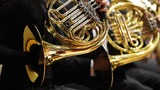Conditional Remix & Share Permitted
CC BY-NC
Rating
0.0 stars

Rate

Type of Unit: Concept

Prior Knowledge

Students should be able to:

Solve problems involving all four operations with rational numbers.
Understand quantity as a number used with a unit of measurement.
Solve problems involving quantities such as distances, intervals of time, liquid volumes, masses of objects, and money, and with the units of measurement for these quantities.
Understand that a ratio is a comparison of two quantities.
Write ratios for problem situations.
Make and interpret tables, graphs, and diagrams.
Write and solve equations to represent problem situations.

Lesson Flow

In this unit, students will explore the concept of rate in a variety of contexts: beats per minute, unit prices, fuel efficiency of a car, population density, speed, and conversion factors. Students will write and refine their own definition for rate and then use it to recognize rates in different situations. Students will learn that every rate is paired with an inverse rate that is a measure of the same relationship. Students will figure out the logic of how units are used with rates. Then students will represent quantitative relationships involving rates, using tables, graphs, double number lines, and formulas, and they will see how to create one such representation when given another.

Subject:
Algebra
Mathematics
Material Type:
Unit of Study
Provider:
Pearson